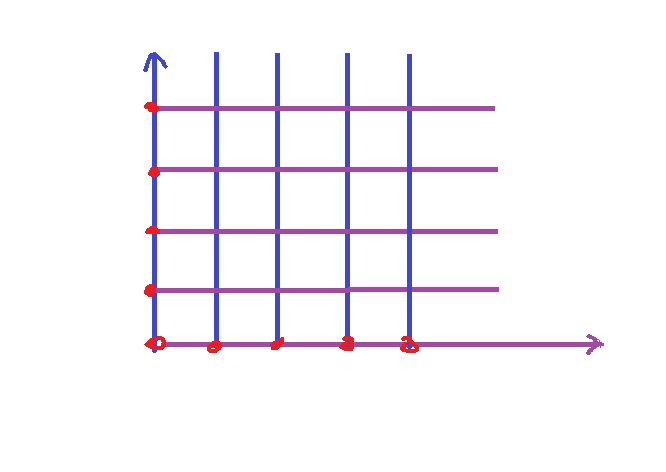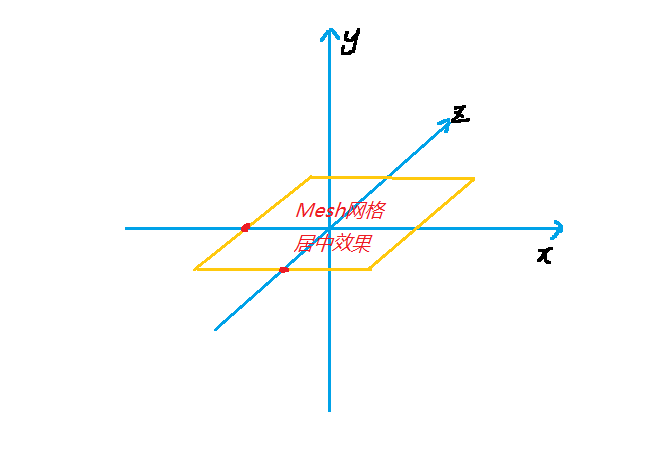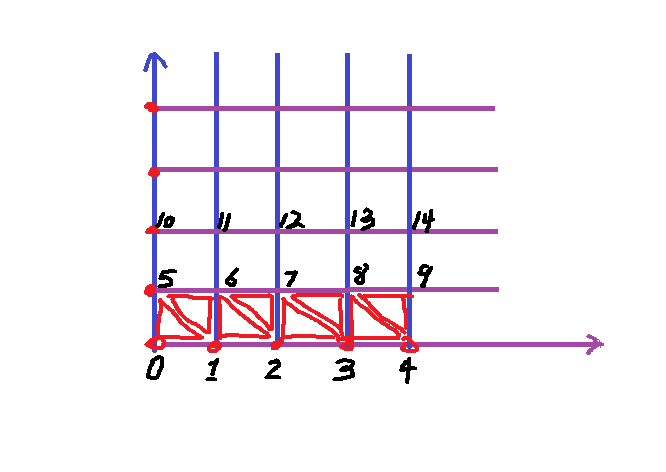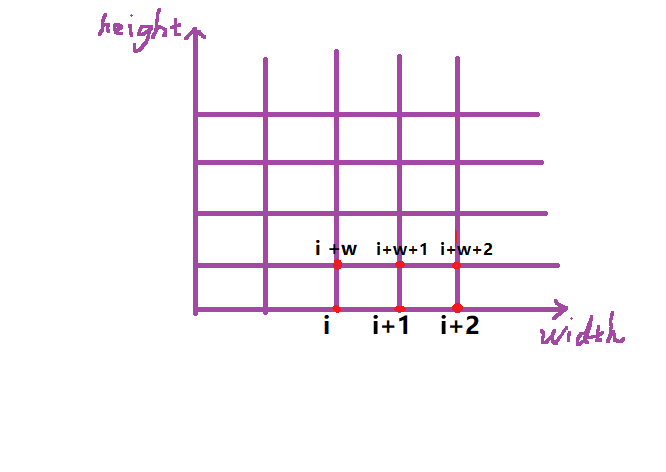public static void GenerateTerrainMesh(float[,] heightMap){
int width =heightMap.GetLength (0);////获取数组第一维元素长度值，赋值给width
int height = heightMap.GetLength(1);////获取数组第二维元素长度值，赋值给height

for (int y=0; y<height; y++){
for (int x =0; x<width; x++){
}
}
}

public class MeshData
{
public Vector3[] vertices;
public int[] triangles;
public MeshData (int meshWidth, int meshHeight)
{  //网格数据存储顶点和三角面信息
vertices = new Vector3[meshWidth * meshHeight];
triangles = new int[(meshWidth - 1) * (meshHeight - 1) * 6];
}
public void AddTriangle(int a, int b, int c)
{ //新建一个添加三角面的方法，a，b，c代替，简化后面的表达式。
triangles[triangleIndex] = a;
triangles[triangleIndex + 1] = b;
triangles[triangleIndex + 2] = c;
triangleIndex += 3;
}
}

public static void GenerateTerrainMesh(float[,] heightMap){
int width =heightMap.GetLength (0);
int height = heightMap.GetLength(1);
//在这继续输入：
MeshData meshData =new MeshData (width,height);
int vertexIndex=0;
//再将遍历循环内加入
meshData.vertices[vertextIndex]=new Vector3(x,heightMap[x,y],y);//将高度图信息存储在(x,z)平面上
vertexIndex ++;public static void GenerateTerrainMesh(float[,] heightMap){

float maxX=(width-1)/2f;
float maxZ=(height-1)/2f;

meshData.vertices[vertextIndex]=new Vector3(x,heightMap[x,y],y);

meshData.vertices[vertexIndex]=new Vector3(x-maxX,heightMap[x,y],y-maxZ);if (x < width -1&& y < height -1)
{//最右侧像素点和上方边界不进行绘制
meshData.AddTriangle(vertexIndex, vertexIndex + width, vertexIndex + 1);
meshData.AddTriangle(vertexIndex + 1, vertexIndex + width, vertexIndex + width + 1);
meshData.AddTriangle(vertexIndex, vertexIndex + width, vertexIndex + 1);
}
vertexIndex++;

public Vector2[] uvs;

MeshData构造里面新增：

uvs =new Vector2[meshWidth*meshHeight];

meshData.uvs[vertexIndex]=new Vector2(x/(float)width,y/(float)height);

public Mesh CreateMesh(){
Mesh mesh = new Mesh();
mesh.vertices=vertices;
mesh.triangles=triangles;
mesh.uv=uvs;
mesh.RecalculateNormals();//重新计算法线，使得所有三角面方向正常显示，从而顺利计算光线反射。
return mesh;//最后传出绘制好顶点，三角面，uv贴图和正确法线分部的mesh信息。

return meshData;

public enum DrawMode{NoiseMap,ColourMap,Mesh};//新增Draw Mesh枚举内容；

else if (drawMode==DrawMode.Mesh){
display.DrawMesh(MeshGenerator.GenerateTerrainMesh(noiseMap),TextureGenerator.TextureFromColourMap(colourMap,mapWidth,mapHeight));//DrawMesh方法我们还没有构建，先写在这里好了。

public MeshFilter meshFilter;//引导mesh信息传递
public MeshRenderer meshRenderer;//mesh信息导入后进行渲染
public void DrawMesh(MeshData meshData , Texture2D texture){  //开始构建绘制mesh方法
meshFilter.sharedMesh=meshData.CreateMesh();
meshRenderer.sharedMaterial.mainTexture=texture;//由于我们可能会在脚本之外会调用修改网格贴图材质，所以这里都采用共用网格和材质类型

Assets里面，Materials文件夹下新建一个材质球，命名Mash Mat；

meshData.vertices[vertexIndex]=new Vector3(x-maxX,heightMap[x,y],y-maxZ);

meshData.vertices[vertexIndex]=new Vector3(maxX-x,heightMap[x,y],maxZ-y);

Last modification：August 12, 2020
If you think my article is useful to you, please feel free to appreciate